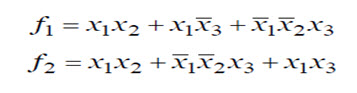# Designing of Sequential Circuits Using PLA

The main drawback of the combinational circuit is, it does not use any memory to save the present and previous states. Hence the previous state of input does not have any effect on the present state of the circuit. Whereas, the sequential circuit has memory so output can vary based on input. This type of circuits uses previous input, output, clock and a memory element. Here the memory elements can be latch or flip-flops. The Sequential circuits are designed by various methods like by using ROMs and flips, PLAs, CPLDs (Complex Programmable Logic Device), FPGAs (Field Programmable Gate Array). In this article, we are going to discuss only on how to design a sequential circuit using PLAs.

The block diagram of the sequential circuit as shown below:

## Designing of Sequential Circuit Using PLAs

Sequential circuits can be realized using PLAs (Programmable Logic Arrays) and flip-flops. In this design, the state assignment may be important because the use of a good state assignment can reduce the required number of product terms and, hence reduce the required size of the PLA. A product term defined as the conjunction of literals, where each literal is either a variable or its negation.

For let us consider the design a code converter. The state table shown below in table can be realized by using one PLA and three flip-flops as shown below figure. This circuit configuration is very similar to ROM flip-flop based design, except that ROM is replaced by the PLA of suitable size. The state assignment leads to the truth table given below. This table could be stored in a PLA with four inputs, 13 product terms, and four outputs, but this would offer little reduced in size as compared with the 16-word ROM.

 X  Q1  Q2  Q3 Z  D1  D2  D3 0    0    0    0 0    0    0    1 0    0    1    0 0    0    1    1 0    1    0    0 0    1    0    1 0    1    1    0 0    1    1    1 1    0    0    0 1    0    0    1 1    0    1    0 1    0    1    1 1    1    0    0 1    1    0    1 1    1    1    0 1    1    1    1 1   0    0    1 1   0    1    1 0   1    0    0 0   1    0    1 1   1    0    1 0   0    0    0 1   0    0    0 X  X   X   X 0   0    1    0 0   1    0    0 1   1    0    0 1   1    0    1 0   1    1    0 1   0    0    0 X   X   X   X X   X   X   X

Table: Truth Table

 PRESENT STATE NEXT STATE X=  0          1 PRESENT OUTPUT (Z) A B         C 1          0 B C D         E E         E 1          0 0          1 D E H          H H         M 0          1 1          0 H M A         A A          – 0          1 1           –

Table: State Table

Here, as there are seven states, three D flip-flops are required. Thus, a PLA circuit with 4 inputs and 4 outputs is required. If the state assignment of the code converter is considered, the resulting output equation and D flip-flop input equations derived from the Karnaugh can be written the following equations

D1= Q1+= Q2”

D2= Q2+= Q2”

D3= Q3+= Q1 Q2 Q3= X” Q1 Q3”= X Q1” Q2”

Z=X” Q3”+ X Q3

 X  Q1  Q2  Q3 Z  D1  D2  D3 –      –    0      ––      1    –      – –      1    1     1 0     1    –      0 1     0    0     – 0     –     –     0 1     –     –      1 0   1    0    0 0   0    1    0 0   0    0    1 0   0    0    1 0   0    0    1 1   0    0    0 1   0    0    0

The PLA table which corresponds to these equations is given in the table above. This table can be realized by using PLA with four inputs, seven product terms, and four outputs. To verify the operation of the above design initially, assume that X=0 and Q1Q2Q3=000. This selects rows – – 0- and 0 – – -0 in the table, so Z=0 and D1D2D3=100. After the active clock edge, Q1Q2Q3=100. If the next input is X=1, then rows – – 0 – and – 1- – are selected, so Z=0 and D1D2D3=110. After the active clock edge, Q1Q2Q3=110.

### Programmable Logic Array (PLA)

Programmable Logic Array is a programmable logical device. It is generally used to implement combinational logic circuits. The PLA has a set of programmable AND planes (AND array), which link to a set of programmable OR planes (OR array), which can then be provisionally complemented to produce an output. This layout allows for a large number of logic functions to be synthesized in the sum of products (SOP) canonical forms. A simple block diagram of a PLA is given below.

The main difference between PLA and PAL (programmable array logic) is,

PLA: Both AND plane and OR plane are programmable.

PAL: Only AND plane is programmable, while OR plane is fixed.

For the better understanding of PLA, here we are considering the below example.

Let’s try to implement these function f1 and f2 are given asInputs x1, x2, x3 and their respective complemented signals are given to programmable AND plane, there we will get AND plane outputs as P1, P2, P3called minterms. Then these signals are given to programmable OR plane to produce required output function f1 and f2 (sum of products). The below figure describes the gate level implementation of the PLA for given functionality.